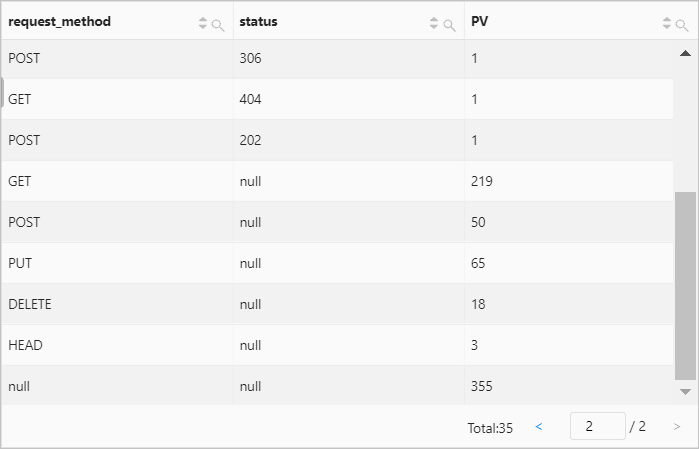The GROUP BY clause is used together with aggregate functions to group analysis results based on one or more columns that you specify. The GROUP BY clause can also be used together with ROLLUP, CUBE, and GROUPING SETS to generate multiple grouping sets.

## Syntax

• GROUP BY

The GROUP BY clause groups analysis results based on one or more columns that you specify.

``````SELECT
key,
...
aggregate function
GROUP BY
key,...``````
• GROUP BY ROLLUP

The GROUP BY ROLLUP clause groups analysis results based on the rollup operation. The clause returns a subtotal for each group and a grand total for all groups. For example, if you use GROUP BY ROLLUP (a, b), the following grouping sets are produced: (a, b), (a, null), and (null, null).

``````SELECT
key,
...
aggregate function
GROUP BY ROLLUP (key,...)``````
• GROUP BY CUBE

The GROUP BY CUBE clause groups analysis results based on all possible combinations of columns. For example, if you use GROUP BY CUBE (a, b), the following grouping sets are produced: (a, b), (null, b), (a, null), and (null, null).

``````SELECT
key,
...
aggregate function
GROUP BY CUBE (key,...)``````
• GROUP BY GROUPING SETS

The GROUP BY GROUPING SETS clause groups analysis results based on the columns that you specify in sequence. For example, if you use GROUP BY GROUPING SETS (a, b), the following grouping sets are produced: (a, null) and (null, b).

``````SELECT
key,
...
aggregate function
GROUP BY GROUPING SETS (key,...)``````
Notice If you use the GROUP BY clause in an analytic statement, the system can query only a column that is included in the GROUP BY clause or perform aggregation on an arbitrary column when the system executes the SELECT statement. For example, `* | SELECT status, request_time, COUNT(*) AS PV GROUP BY status` is invalid because the request_time column is not included in the GROUP BY clause. You can change the statement to `* | SELECT status, arbitrary(request_time), count(*) AS PV GROUP BY status`, which is valid.

## Parameters

Parameter Description
key The name of the log field or the name of the column whose values are returned by an aggregate function. The GROUP BY clause groups results based on the log field or column that you specify.

The GROUP BY clause allows you to specify one or more columns.

aggregate function The aggregate function that is used together with the GROUP BY clause. The GROUP BY clause is often used together with aggregate functions, such as min, max, avg, sum, and count. For more information, see Aggregate function.

## Examples

### Example 1

Group the numbers of page views (PVs) based on status codes.

• Query statement
``* | SELECT status, count(*) AS PV GROUP BY status``
• Query and analysis results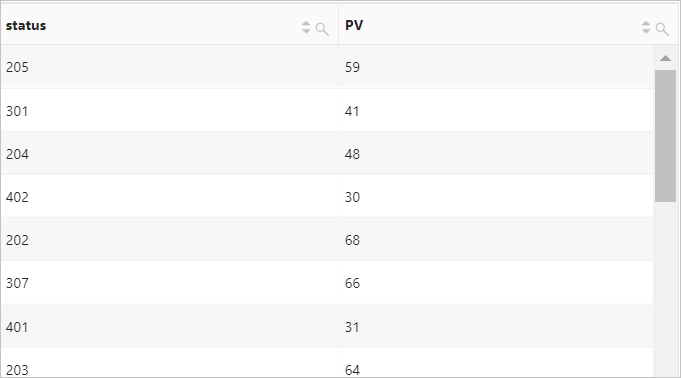### Example 2

Group the numbers of PVs based on 1-hour intervals. In the following statement, the __time__ field is a reserved field in Log Service. This field indicates the time column. time is the alias of `date_trunc('hour', __time__)`. For more information about the date_trunc function, see date_trunc function.

• Query statement
``````* |
SELECT
count(*) AS PV,
date_trunc('hour', __time__) AS time
GROUP BY
time
ORDER BY
time
LIMIT
1000                       ``````
• Query and analysis results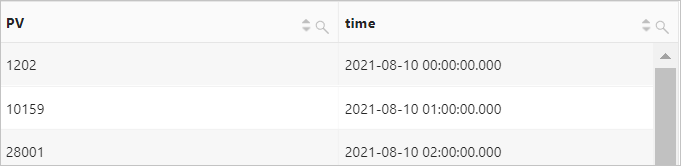### Example 3

Group the numbers of PVs based on 5-minute intervals.

• Query statement

The date_trunc function allows you to measure statistics only based on a fixed interval. The interval is determined by the time unit that you specify in the function. If you want to measure statistics based on a custom interval, we recommend that you perform a mathematical modulo operation to group data. In the following statement, %300 specifies that a mathematical modulo operation is performed to group data at 5-minute intervals.

``````* |
SELECT
count(*) AS PV,
__time__-__time__ % 300 AS time
GROUP BY
time
LIMIT
1000``````
• Query and analysis results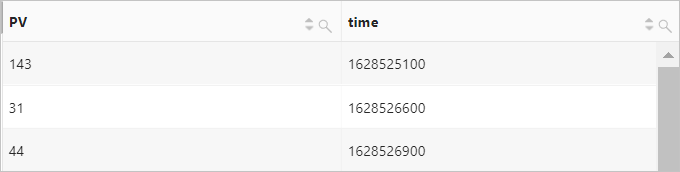### Example 4

Group the numbers of PVs based on the request_method and status columns. The GROUP BY GROUPING SETS clause calculates the numbers of PVs first for each request method and then for each status.

• Query statement
``````* |
SELECT
request_method,
status,
count(*) AS PV
GROUP BY
GROUPING SETS (request_method, status)``````
• Query and analysis results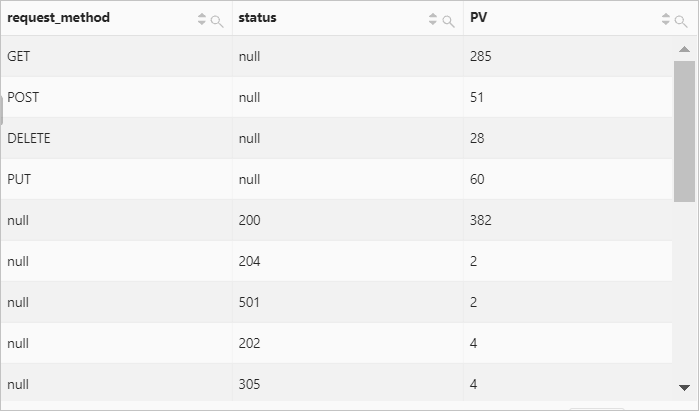### Example 5

Group the numbers of PVs based on the request_method and status columns. The following grouping sets are produced: (null, null), (request_method, null), (null, status), and (request_method, status). The GROUP BY CUBE clause calculates the numbers of PVs for each group.

• Query statement
``````* |
SELECT
request_method,
status,
count(*) AS PV
GROUP BY
CUBE (request_method, status)``````
• Query and analysis results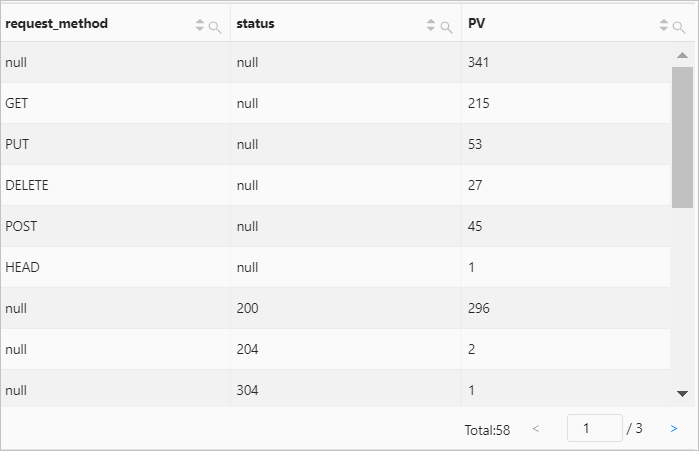### Example 6

Group the numbers of PVs based on the request_method and status columns. The following grouping sets are produced: (request_method, status), (request_method, null), and (null, null). The GROUP BY ROLLUP clause calculates the numbers of PVs for each group.

• Query statement
``````* |
SELECT
request_method,
status,
count(*) AS PV
GROUP BY
ROLLUP (request_method, status)``````
• Query and analysis results